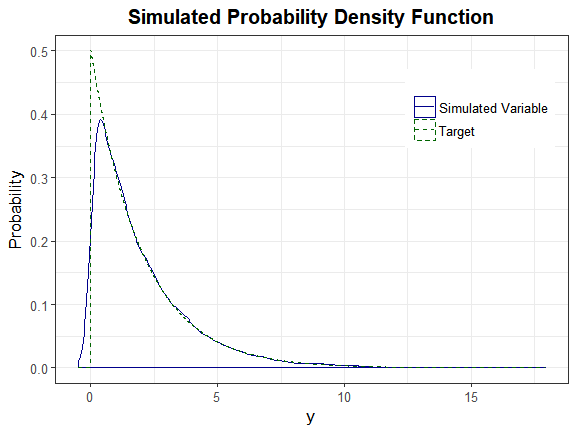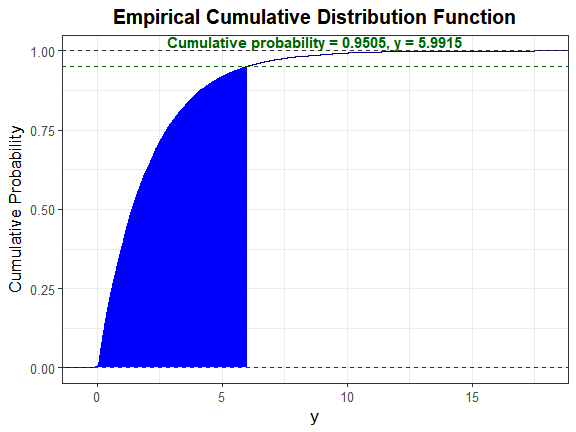# Simulation of Correlated Data with Multiple Variable Types

Generate continuous (normal or non-normal), binary, ordinal, and count (Poisson or Negative Binomial) variables with a specified correlation matrix. It can also produce a single continuous variable. This package can be used to simulate data sets that mimic real-world situations (i.e. clinical or genetic data sets, plasmodes). All variables are generated from standard normal variables with an imposed intermediate correlation matrix. Continuous variables are simulated by specifying mean, variance, skewness, standardized kurtosis, and fifth and sixth standardized cumulants using either Fleishman's third-order () or Headrick's fifth-order () polynomial transformation. Binary and ordinal variables are simulated using a modification of the ordsample() function from 'GenOrd'. Count variables are simulated using the inverse cdf method. There are two simulation pathways which differ primarily according to the calculation of the intermediate correlation matrix. In Correlation Method 1, the intercorrelations involving count variables are determined using a simulation based, logarithmic correlation correction (adapting Yahav and Shmueli's 2012 method, ). In Correlation Method 2, the count variables are treated as ordinal (adapting Barbiero and Ferrari's 2015 modification of GenOrd, ). There is an optional error loop that corrects the final correlation matrix to be within a user-specified precision value of the target matrix. The package also includes functions to calculate standardized cumulants for theoretical distributions or from real data sets, check if a target correlation matrix is within the possible correlation bounds (given the distributions of the simulated variables), summarize results (numerically or graphically), to verify valid power method pdfs, and to calculate lower standardized kurtosis bounds.

# SimMultiCorrData

The goal of `SimMultiCorrData` is to generate continuous (normal or non-normal), binary, ordinal, and count (Poisson or Negative Binomial) variables with a specified correlation matrix. It can also produce a single continuous variable. This package can be used to simulate data sets that mimic real-world situations (i.e. clinical data sets, plasmodes, as in Vaughan et al., 2009). All variables are generated from standard normal variables with an imposed intermediate correlation matrix. Continuous variables are simulated by specifying mean, variance, skewness, standardized kurtosis, and fifth and sixth standardized cumulants using either Fleishman's Third-Order or Headrick's Fifth-Order Polynomial Transformation. Binary and ordinal variables are simulated using a modification of `GenOrd::ordsample`. Count variables are simulated using the inverse cdf method. There are two simulation pathways which differ primarily according to the calculation of the intermediate correlation matrix `Sigma`. In Correlation Method 1, the intercorrelations involving count variables are determined using a simulation based, logarithmic correlation correction (adapting Yahav and Shmueli's 2012 method). In Correlation Method 2, the count variables are treated as ordinal (adapting Barbiero and Ferrari's 2015 modification of `GenOrd`). There is an optional error loop that corrects the final correlation matrix to be within a user-specified precision value. The package also includes functions to calculate standardized cumulants for theoretical distributions or from real data sets, check if a target correlation matrix is within the possible correlation bounds (given the distributions of the simulated variables), summarize results (numerically or graphically), to verify valid power method pdfs, and to calculate lower standardized kurtosis bounds.

There are several vignettes which accompany this package that may help the user understand the simulation and analysis methods.

1. Benefits of SimMultiCorrData and Comparison to Other Packages describes some of the ways `SimMultiCorrData` improves upon other simulation packages.

2. Variable Types describes the different types of variables that can be simulated in `SimMultiCorrData`.

3. Function by Topic describes each function, separated by topic.

4. Comparison of Correlation Method 1 and Correlation Method 2 describes the two simulation pathways that can be followed.

5. Overview of Error Loop details the algorithm involved in the optional error loop that improves the accuracy of the simulated variables' correlation matrix.

6. Overall Workflow for Data Simulation gives a step-by-step guideline to follow with an example containing continuous (normal and non-normal), binary, ordinal, Poisson, and Negative Binomial variables. It also demonstrates the use of the standardized cumulant calculation function, correlation check functions, the lower kurtosis boundary function, and the plotting functions.

7. Comparison of Simulation Distribution to Theoretical Distribution or Empirical Data gives a step-by-step guideline for comparing a simulated univariate continuous distribution to the target distribution with an example.

8. Using the Sixth Cumulant Correction to Find Valid Power Method Pdfs demonstrates how to use the sixth cumulant correction to generate a valid power method pdf and the effects this has on the resulting distribution.

## Installation instructions

`SimMultiCorrData` can be installed using the following code:

## Example

This is a basic example which shows you how to solve a common problem: Compare a simulated exponential(mean = 2) variable to the theoretical exponential(mean = 2) density.

### Step 1: Obtain the standardized cumulants

In R, the exponential parameter is rate <- 1/mean.

### Step 2: Simulate the variable

Note that `calc_theory` returns the standard deviation, not the variance. The simulation functions require variance as the input.

### Step 4: Select a critical value

Let alpha = 0.05.

### Step 5: Solve for \$\Large z'\$

Since the exponential(2) distribution has a mean and standard deviation equal to 2, solve \$\Large 2 * p(z') + 2 - y_star = 0\$ for \$\Large z'\$. Here, \$\Large p(z') = c0 + c1 * z' + c2 * z'^2 + c3 * z'^3 + c4 * z'^4 + c5 * z'^5\$.

### Step 6: Calculate \$\Large \Phi(z')\$

This is approximately equal to the alpha value of 0.05, indicating the method provides a good approximation to the actual distribution.

### Step 7: Plot graphsWe can also plot the empirical cdf and show the cumulative probability up to y_star.# SimMultiCorrData 0.2.2

1. Fixed error in formation of intermediate matrices with `findintercorr2`.
2. Fixed check for repeated continuous distribution when using `method` = "Fleishman".
3. Updated citations.
4. Fixed function/package links in documentation.
5. Added automated testing with 'testthat' package.

# SimMultiCorrData 0.2.1

1. Updated `calc_theory()` and plotting functions which call it to permit pdf specified by `fx`, `lower`, and `upper`.
2. Adjusted `rcorrvar()` and `rcorrvar2()` summary of continuous variables when using `method = "Fleishman"`.
3. Fixed error in `rcorrvar()`, `rcorrvar2()`, `valid_corr()`, `valid_corr2()`, and `error_loop()` to permit 0 or 1 continuous variables.
4. Updated `calc_lower_skurt()` for case of non-convergence when applying `Six` vector with `method = "Polynomial"`.
5. Added `lower` and `upper` parameters to `plot_cdf()` to use as inputs for `cdf_prob()`.

# SimMultiCorrData 0.2.0

1. Fixed error in `findintercorr2()` so now you can generate 1 ordinal variable using correlation method 2 (with `rcorrvar2()`).
2. Fixed error in `chat_nb()` so you can use `size` (success probability) and `mu` (mean) parameters for Negative Binomial variables when using correlation method 1 (with `rcorrvar1()`).
3. Updated `find_constants()` and `calc_lower_skurt()` (to remove duplicate rows in solutions before executing `pdf_check()`) in order to decrease computation time.
4. Updated `rcorrvar()`, `rcorrvar2()`, `valid_corr()`, and `valid_corr2()` to check for identical continuous distributions before calculating the power method constants in order to decrease computation time. If a distribution is repeated, the constants are only calculated once.
5. Made the following updates to `error_loop()` and `error_vars()`:
• all variables are regenerated in each iteration and the final correlation is calculated each time and at the end with all variables (instead of pairwise)
• eigenvalue decomposition on `Sigma` is done using the maximum of 0 and the eigenvalues (in case `Sigma` is not positive-definite and the eigenvalues are negative); this replaces the use of `Matrix::nearPD()`
• update function is based on the correlation calculated from the previous iteration instead of the pre-error loop final correlation.
• fixed `ifelse()` statement in choice of update function (affects negative correlations only)
1. Updated the Overview of Error Loop vignette to reflect above changes.
2. Fixed `ifelse()` statement in choice of update function for `ordnorm()` (affects negative correlations only).
3. Made the following updates to `calc_theory()`:
• `params` input accepts up to 4 parameters
• 39 distributions are available by name (as `Dist` input)
1. Made the following updates to `plot_pdf_theory()`, `plot_sim_pdf_theory()`, and `plot_sim_theory()`:
• `params` input accepts up to 4 parameters
• 39 distributions are available by name (as `Dist` input) plus Poisson and Negative Binomial for `plot_sim_pdf_theory()` and `plot_sim_theory()`
1. Added extra `ggplot2` parameters to the graphing functions to allow control over the appearance of the legend, axes labels and titles, and plot title.
2. Changed the example in the Overall Workflow for Data Simulation vignette.
3. Changed the examples in the `rcorrvar()` and `rcorrvar2()` documentation.
4. Updated documentation to some of the functions.

# SimMultiCorrData 0.1.0

Initial package release.

# Reference manual

install.packages("SimMultiCorrData")

0.2.2 by Allison Cynthia Fialkowski, 3 years ago

https://github.com/AFialkowski/SimMultiCorrData

Browse source code at https://github.com/cran/SimMultiCorrData

Authors: Allison Cynthia Fialkowski

Documentation:   PDF Manual